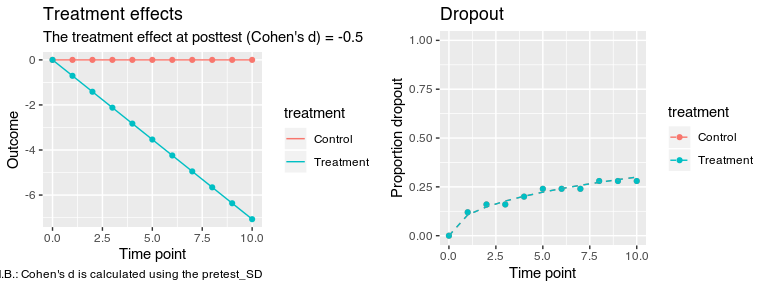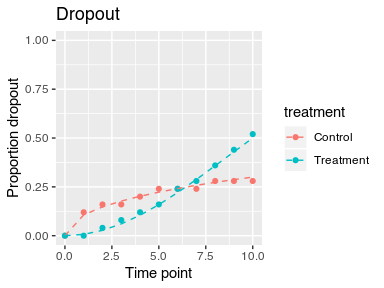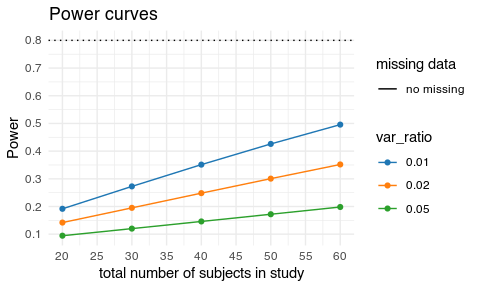Introduction

This vignette shows how to use the powerlmm-package to design a two-level longitudinal study. The package does not only contain tools to calculate power for these models, but also tools to help you understand the implications of the model’s parameters. The real world performance of the study design can also be evaluated using Monte Carlo simulations (see the simulation vignette). The package also includes functions to help you understand the consequences of ignoring clustering effects at the third level (see the three-level vignette). The design effect can be calculated, as well as the inflation of Type I errors when a random slope at the third level is ignored.

Supported models

Currently power can be calculated for the following designs

• Two-level model (subjects with repeated measures)
• Nested three-level models (subjects with repeated measures, nested within e.g. therapists)
• Partially nested designs (only one treatment arm in the nested three-level models have clustering)
• Random slopes: It is possible to include random slopes at both the cluster and subject level.
• Unbalanced designs: Unbalanced designs are supported; e.g. treatments can have different number of subjects and/or clusters. It is also possible to have unbalanced cluster sizes within a treatment.
• Missing data: The power calculations can account for missing data, either by manually specifying the dropout per time point, or by using a Weibull survival function.

Two-level longitudinal linear mixed-effects model with random slopes

This is the typical longitudinal linear mixed model, which in multilevel notion is written as

\begin{align} \text{Level 1}& \notag\\\ Y_{ij} &= \beta_{0j} + \beta_{1j}t_{ij} + R_{ij}\\\ \text{Level 2}& \notag\\\ \beta_{0j} &= \gamma_{00} + \gamma_{01} TX_j + U_{0j} \\\ \beta_{1j} &= \gamma_{10} + \gamma_{11} TX_j + U_{1j} \\\ \end{align} with, $\begin{equation} \begin{pmatrix} U_{0j} \\\ U_{1j} \end{pmatrix} \sim\mathcal{N} \left( \begin{matrix} 0 &\\\ 0 \end{matrix} , \begin{matrix} \sigma_{u_0}^2 & \sigma_{u_{01}}\\\ \sigma_{u_{01}} & \sigma_{u_1}^2 \end{matrix} \right) , \end{equation}$ and $\begin{equation} R_{ij} \sim\mathcal{N}(0, ~\sigma_e^2) \end{equation}$

All designs are setup using the study_parameters function. Parameters can be specified either directly or by their standardized counterpart.

The arguments in study_parameters are

parameter study_parameters()-argument
$$\gamma_{00}$$ fixed_intercept
$$\gamma_{01}$$ NA; assumed to be 0
$$\gamma_{10}$$ fixed_slope
$$\gamma_{11}$$ calculated from effect_size
$$\sigma_{u_0}$$ sigma_subject_intercept
$$\sigma_{u_1}$$ sigma_subject_slope
$$\sigma_{u_{01}}$$ calculated from cor_subject
$$\sigma_e$$ sigma_error

Standardized and unstandardized formulation

For a two-level model power depends on n1, n2, the amount of baseline variance at the subject level (icc_pre_subjects) and the ratio of subject-level random slope variance to the within-subject error variance (var_ratio).

Standardized Calculation
icc_pre_subjects $$\sigma_{u_0}^2/(\sigma_{u_0}^2 + \sigma_e^2)$$
var_ratio $$\sigma_{u_1}^2/\sigma_e^2$$

Effect sizes

The argument effect_size either accepts the raw difference between the groups at posttest, or a standardized effect size by passing the cohend(...) function. Cohen’s d can be calculated using either the pretest, posttest, or random slope SD as the standardizer (denominator). See ?cohend for options.

For standardized effect sizes that use either the pretest or posttest SD, the effect size refers to the standardized difference between the groups at posttest, $\delta_{101} = \frac{\text{ES} \times \sigma}{T_{end}}.$ Where the standardizer $$\sigma$$ is one of the following standardizers (based either on the treatment or control groups variance components):

• pretest SD, $\sigma_{pre} = \sqrt{\sigma_{u0}^2 + \sigma_{e}^2}.$
• posttest SD, $\sigma_{post} = \sqrt{\sigma_{u0}^2 + 2T_{end}\sigma_{u01} + T_{end}^2\sigma_{u1}^2 + \sigma_{e}^2}.$

If the random slope SD (cf. Raudenbush and Xiao-Feng (2001)) is used as the standardizer, the ES now indicate the difference per unit of time, $\delta_{101} = \text{ES} \times \sigma_{u1}.$

Setting up the model using study_parameters()

Here’s an example of specifying the “same” model using standardized or unstandardized inputs.

p1 <- study_parameters(n1 = 11,
n2 = 25,
sigma_subject_intercept = 1.44,
sigma_subject_slope = 0.2,
sigma_error = 1.44,
effect_size = cohend(-0.5,
standardizer = "pretest_SD"))

p2 <- study_parameters(n1 = 11,
n2 = 25,
icc_pre_subject = 0.5,
var_ratio = 0.019,
effect_size = cohend(-0.5,
standardizer = "pretest_SD"))
p1
##
##      Study setup (two-level)
##
##               n1 = 11
##               n2 = 25 (treatment)
##                    25 (control)
##                    50 (total)
##          dropout = No missing data
## icc_pre_subjects = 0.5
##        var_ratio = 0.02
##      effect_size = -0.5 (Cohen's d [SD: pretest_SD])

To calculate power we use get_power

get_power(p2)
##
##      Power Analysis for Longitudinal Linear Mixed-Effects Models
##             with missing data and unbalanced designs
##
##               n1 = 11
##               n2 = 25 (treatment)
##                    25 (control)
##                    50 (total)
##          dropout = No missing data
## icc_pre_subjects = 0.5
##        var_ratio = 0.02
##      effect_size = -0.5 (Cohen's d [SD: pretest_SD])
##               df = 48
##            alpha = 0.05
##            power = 31 %

Missing data

Missing data can be accounted for in the power calculations by the argument dropout. Intermittent missing data is not currently supported, thus missing data is monotonically increasing. Two helper functions are used to define the dropout pattern; either dropout_manual or dropout_weibull. Here I will use dropout_weibull.

p2 <- study_parameters(n1 = 11,
n2 = 25,
icc_pre_subject = 0.5,
var_ratio = 0.019,
dropout = dropout_weibull(proportion = 0.3,
rate = 1/2),
effect_size = cohend(-0.5,
standardizer = "pretest_SD"))

Here I’ve chosen to have a total of 30 % of the participant dropout during the study, with more dropout occurring earlier in the study period. We can plot the model and missing data pattern using plot.

plot(p2)And the power can be calculated using get_power.

get_power(p2)
##
##      Power Analysis for Longitudinal Linear Mixed-Effects Models
##             with missing data and unbalanced designs
##
##               n1 = 11
##               n2 = 25 (treatment)
##                    25 (control)
##                    50 (total)
##          dropout =  0,  1,  2,  3,  4,  5,  6,  7,  8,  9, 10 (time)
##                     0, 11, 15, 18, 20, 22, 24, 26, 27, 29, 30 (%, control)
##                     0, 11, 15, 18, 20, 22, 24, 26, 27, 29, 30 (%, treatment)
## icc_pre_subjects = 0.5
##        var_ratio = 0.02
##      effect_size = -0.5 (Cohen's d [SD: pretest_SD])
##               df = 48
##            alpha = 0.05
##            power = 25 %

Not surprisingly, power is decreased compared to the model with no missing data.

Different dropout patterns per treatment group

The helper function per_treatment allows some options to differ by treatment arm, here we will use it to specify a different dropout pattern in each treatment group.

d <- per_treatment(control = dropout_weibull(proportion = 0.3,
rate = 1/2),
treatment = dropout_weibull(proportion = 0.5,
rate = 2))

p2 <- study_parameters(n1 = 11,
n2 = 25,
icc_pre_subject = 0.5,
var_ratio = 0.019,
dropout = d,
effect_size = cohend(-0.5,
standardizer = "pretest_SD"))

plot(p2, type = "dropout")Different number of subjects per treatment group

The amount of subjects per treatment group can also be specified per treatment. Let’s specify two studies that both have a total of 60 participants. The first have equal allocation (30 per group), and the second unequal allocation.

p1 <- study_parameters(n1 = 11,
n2 = 30,
icc_pre_subject = 0.5,
var_ratio = 0.019,
effect_size = cohend(-0.5,
standardizer = "pretest_SD"))
p2 <- study_parameters(n1 = 11,
n2 = per_treatment(control = 10,
treatment = 50),
icc_pre_subject = 0.5,
var_ratio = 0.019,
effect_size = cohend(-0.5,
standardizer = "pretest_SD"))

p1
##
##      Study setup (two-level)
##
##               n1 = 11
##               n2 = 30 (treatment)
##                    30 (control)
##                    60 (total)
##          dropout = No missing data
## icc_pre_subjects = 0.5
##        var_ratio = 0.02
##      effect_size = -0.5 (Cohen's d [SD: pretest_SD])
p2
##
##      Study setup (two-level)
##
##               n1 = 11
##               n2 = 50 (treatment)
##                    10 (control)
##                    60 (total)
##          dropout = No missing data
## icc_pre_subjects = 0.5
##        var_ratio = 0.02
##      effect_size = -0.5 (Cohen's d [SD: pretest_SD])

If we calculate power we can see that balanced allocation is more powerful.

get_power(p1)$power ##  0.3622166 get_power(p2)$power
##  0.2237726

Power curves

Since power is influenced by many different parameters, the function get_power_table lets you compare the effect of up to 3 different parameters, and visualize the relationships. Let’s see how the number of subjects and the variance ratio influences power.

p1 <- study_parameters(n1 = 11,
n2 = 30,
icc_pre_subject = 0.5,
var_ratio = 0.019,
effect_size = cohend(-0.5,
standardizer = "pretest_SD"))

x <- get_power_table(p1, n2 = seq(10, 30, by = 5), var_ratio = c(0.01, 0.02, 0.05))
x
##    n2 var_ratio      power tot_n    dropout
## 1  10      0.01 0.19168927    20 no missing
## 2  15      0.01 0.27261679    30 no missing
## 3  20      0.01 0.35125795    40 no missing
## 4  25      0.01 0.42599746    50 no missing
## 5  30      0.01 0.49575126    60 no missing
## 6  10      0.02 0.14196723    20 no missing
## 7  15      0.02 0.19511492    30 no missing
## 8  20      0.02 0.24823202    40 no missing
## 9  25      0.02 0.30066036    50 no missing
## 10 30      0.02 0.35184744    60 no missing
## 11 10      0.05 0.09453344    20 no missing
## 12 15      0.05 0.12011950    30 no missing
## 13 20      0.05 0.14598735    40 no missing
## 14 25      0.05 0.17204597    50 no missing
## 15 30      0.05 0.19819649    60 no missing
plot(x)We see that the variance ratio influences power more than the number of subjects.